## Example Questions

### Example Question #3 : Operations

Solve for: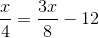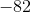Explanation:

Begin by isolating thefactors: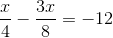Now, the common denominator of these two fractions is.  Therefore, multiply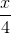by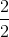: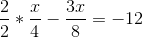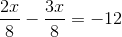Now, you can subtract the left values: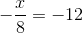Now, multiply both sides by: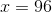### Example Question #4 : Operations

Simplify: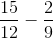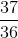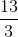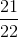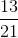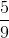Explanation:

Just like adding fractions, when you subtract fractions, you need to find a common denominator. Forand, the least common denominator is. In order to do your subtraction, you need to multiply appropriately to give your fractions this denominator: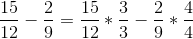Which is the same as...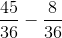Now, you can subtract the numerators and retain the denominator:### Example Question #1 : How To Subtract Fractions

Which of the following is true?

Quantity A: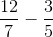Quantity B: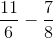Quantity A is larger.

The two quantities are equal.

The relationship between the quantities cannot be determined.

Quantity B is larger.

Quantity A is larger.

Explanation:

First, consider each quantity separately.

Quantity AThese two fractions do not have a common factor. Their common denominator is. Thus, we multiply the fractions as follows to give them a common denominator: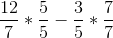This is the same as: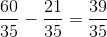Quantity BThe common denominator of these two values is.  Therefore, you multiply the fractions as follows to give them a common denominator: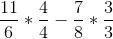This is the same as: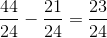Since Quantity A is larger thanand Quantity B is a positive fraction less than, we know that Quantity A is larger without even using a calculator.